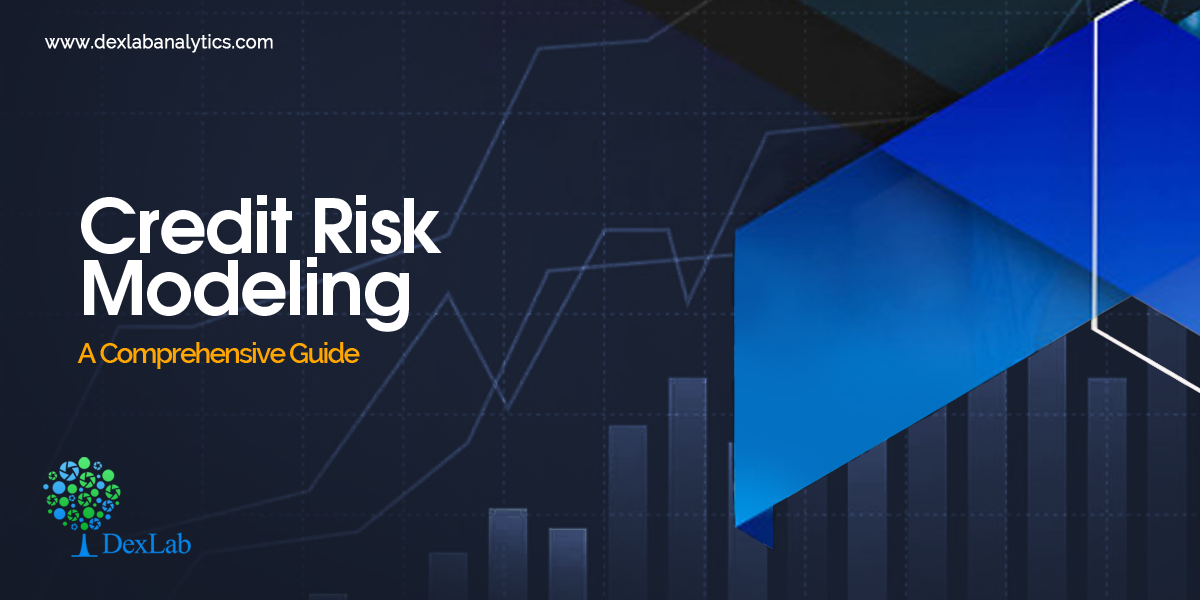# Credit Risk Modeling: A Comprehensive Guide

## Recent PostsCredit Risk Modeling is the analysis of the credit risk of a borrower. It helps in understanding the risk, which a lender may face when he offers a credit.

#### What is Credit Risk?

Credit risk is the risk involved in any kind of loan. In other words, it is the risk that a lender runs when he lends a sum to somebody. It is thus, the risk of not getting back the principal sum or the interests of it on time.
Suppose, a person is lending a sum to his friend, then the credit risk models will help him to assess the probability of timely payments and estimate the total loss in case of defaulters.

#### Credit Risk Modelling and its Importance

In the fast-paced world of now, a loss cannot be afforded at any cost. Here’s where the Credit Risk Modeling steps in. It primarily benefits the lenders by accurate approximation of the credit risk of a borrower and thereby, cutting the losses short.

Credit Risk Modelling is extensively used by financial institutions around the world to estimate the credit risk of potential borrowers. It helps them in calculating the interest rates of the loans and also deciding on whether they would grant a particular loan or not.

#### The Changing Models for the Analysis of Credit Risks

With the rapid progress of technology, the traditional models of credit risks are giving way to newer models using R and Python. Moreover, credit risk modeling using the state-of-the-art tools of analytics and Big Data are gaining huge popularity.

Along with the changing technology, the advancing economies and the successive emergence of a range of credit risks have also transformed the credit risk models of the past.

#### What Affects Credit Risk Modeling?

A lender runs a varying range of risks from disruption of cash flows to a hike in the collection costs, from the loss of interest/interests to losing the whole sum altogether. Thus, Credit Risk Modelling is paramount in importance at this age we are living. Therefore, the process of assessing credit risk should be as exact as feasible.

However, in this process, there are 3 main factors that regulate the risk of the credit of the borrowers. Here they are:

1. The Probability of Default (PD) – This refers to the possibility of a borrower defaulting a loan and is thus, a significant factor to be considered when modeling credit risks. For the individuals, the PD score is modeled on the debt-income ratio and existing credit score. This score helps in figuring out the interest rates and the amount of down payment.
2. Loss Given Default (LGD) – The Loss Given Default or LGD is the estimation of the total loss that the lender would incur in case the debt remains unpaid. This is also a critical parameter that you should weigh before lending a sum. For instance, if two different borrowers are borrowing two different sums, the credit risk profiles of the borrower with a large sum would vary greatly to the other, who is borrowing a much smaller sum of money, even though their credit score and debt-income ratio match exactly with each other.
3. Exposure at Default (EAD) – EAD helps in calculating the total exposure that a lender is subjected to at any given point in time. This is also a significant factor exposing the risk appetite of the lender, which considerably affects the credit risk.#### Endnotes

Though credit risk assessment seems like a tough job to assume the repayment of a particular loan and its defaulters, it is a peerless method which will give you an idea of the losses that you might incur in case of delayed payments or defaulters.

.### Call us to know more### Gurgaon+91 931 572 5902